# DPL 9 Help

Gamma Distribution

#Gamma Distribution

The Gamma Distribution can be used to represent the waiting time until the nth occurrence of an event, assuming a constant occurrence rate per unit time. Some examples are the time to the arrival of a fixed number of vehicles (to a given location) or the time to failure of a system designed to accept a fixed number of overloads before failing.

The Gamma Distribution is defined for all positive values of x. It includes both a power of x and a term that declines exponentially. Its parameters are a and b, both of which must be positive. The distribution is: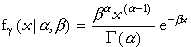where x>0, a>0, and b>0. The gamma function,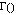, is a generalization of the factorial function. Factorials are only defined for integers, but the gamma function can have any number for an argument. If n is an integer,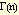= (n-1)!.

The equations for the mean and variance are: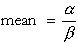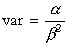The equations for the parameters are: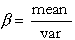The mean must be positive.

Versions: DPL Professional, DPL Enterprise, DPL Portfolio